Arduino Keypad: Interfacing with 4x4 Matrix

Using a keypad is an upgrade over using buttons for input on your Arduino project. Actually, a keypad is a set of 12 or 16 buttons wired so that the pin usage is reduced. In this tutorial, I will explain how a keypad matrix works and how to use it with an Arduino.

As shown in my arduino led control tutorial, wiring one button to the Arduino requires using one of its pins: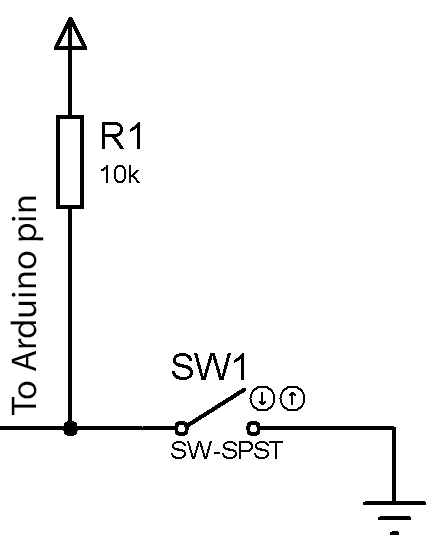So if you would need 16 different buttons, you may need to use 16 Arduino pins. However, a keypad matrix is wired differently: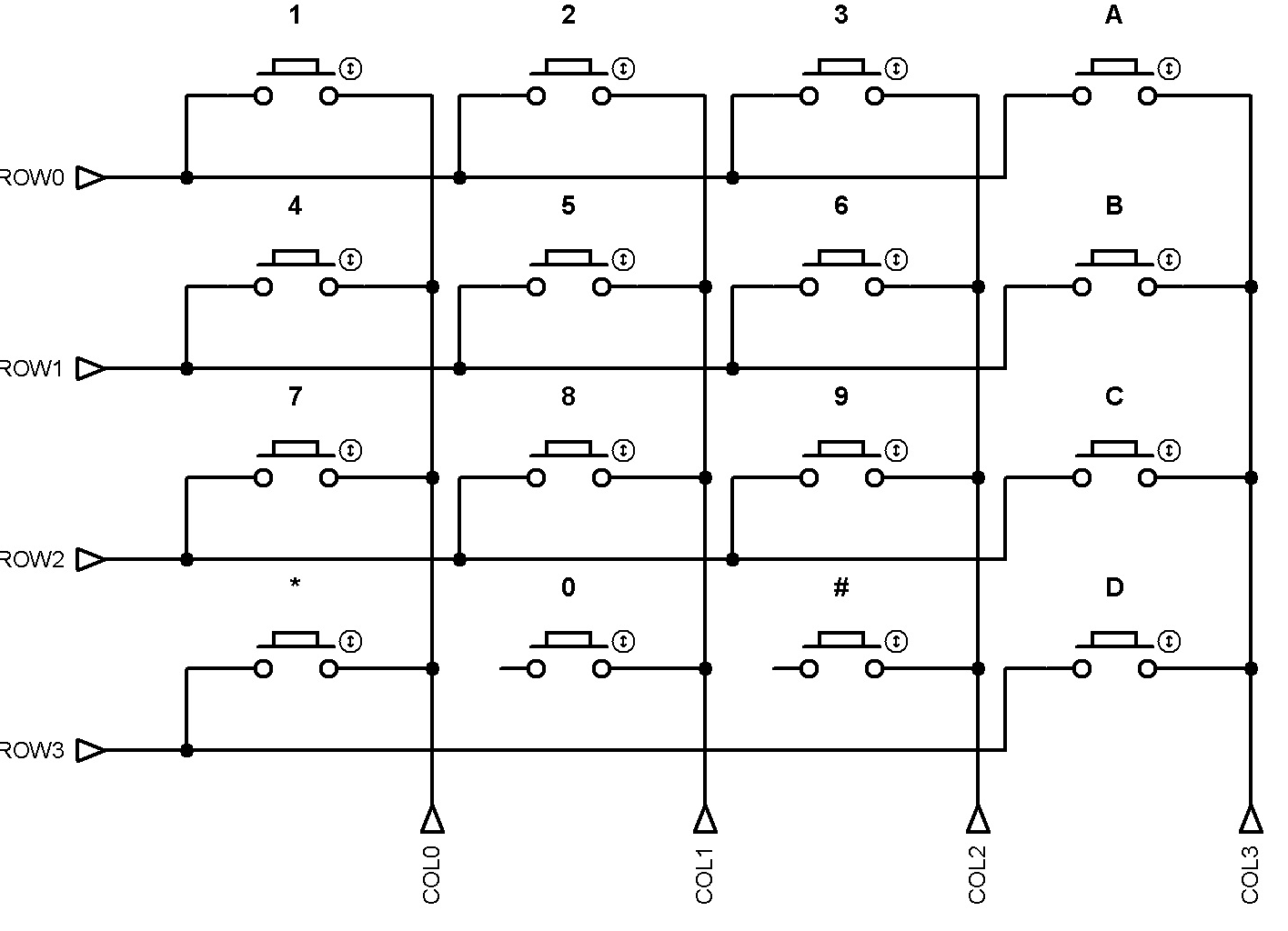A 4x4 keypad matrix has 8 pins divided into 4 rows and 4 columns. When a button is pressed, one row pin will be shorted out with a column pin. For example, if you press button “1”, row “0” will be connected to column “0”.

How a Keypad Matrix Works

Now to be able to make the keypad matrix work with an Arduino, we just need to check which row and column is connected. This may sound easy but it’s not. The most common way to implement this by making a column pin high while making the rest of the columns low and do that in sequence like a LED chaser.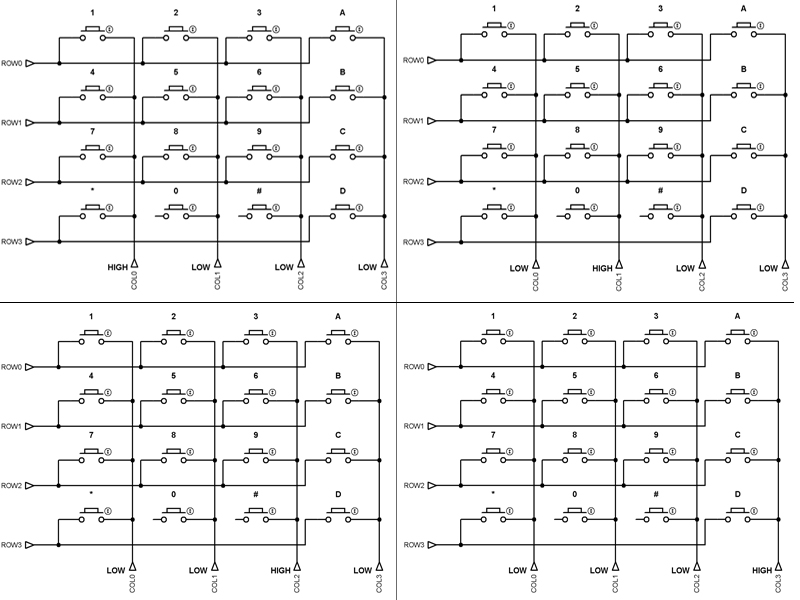Consider the first image (upper left) on the panel above where column “0” is high and the rest of the columns are low. If you press the “1” button, row “0” will be high because by then it will be connected to column “0”. So if you scan row “0” using digitalWrite() while column “0” is high, this means the user pressed button “1”. To detect every button that is pressed, we also need to scan each rows individually. So basically, you can summarize the process like this: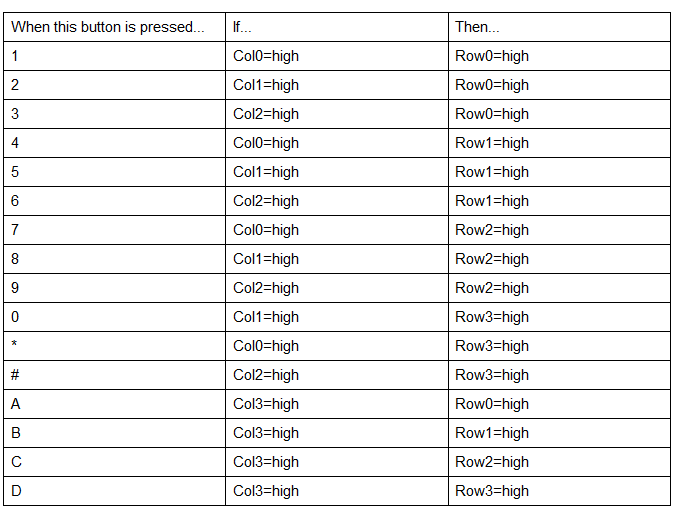Keypad Scanning Arduino Sketch

Now we can implement the scanning technique I described above on an Arduino sketch. By the way, I’ll be using this 4x4 matrix keypad for this example and the rest of this article:To continue, we will use this wiring diagram: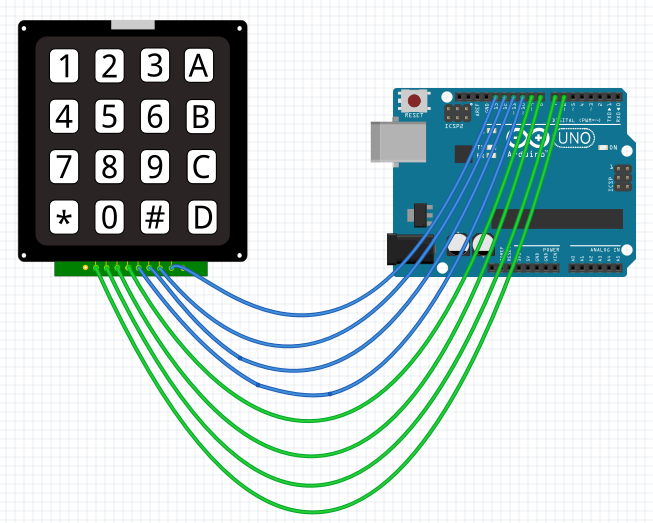Here, the rows are connected to digital pins 10 to 13 while the columns pins are connected to digital pins 6 to 9.

Here is now the Arduino sketch:

Using the Keypad Library

The sketch above is quite long. Isn’t there a quicker way? Yes there is and that’s by using the official Arduino Keypad library.

Although it’s official, the Keypad library is not installed on the IDE by default. To install the library, go to Sketch > Include Library > Manage Libraries. Type “keypad” on the search box and locate the library by Mark Stanley and Alexander Brevig.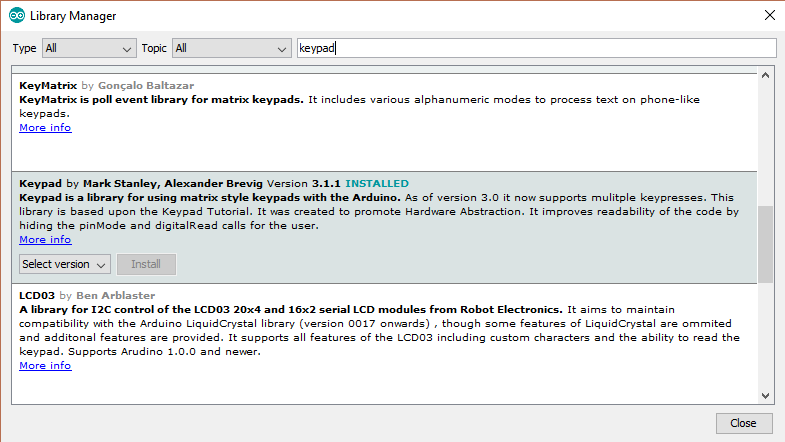Once installed, the Keypad folder should now appear on the examples menu. There are a lot of examples provided in this folder. Here is the CustomKeyboard sketch:

I’ve modified the sketch to fit the wiring diagram we have above. The output of this sketch is the same as the longer sketch I’ve previously gave.

The above sketch is way shorter than the one I’ve provided. And since you can create a Keypad class using the library, you can even make multiple keypads, provided your Arduino still have available pins. I highly recommend using this Keyboard library.

Using Fewer Pins with Keypad Matrix

There is another way to use the 4x4 keypad matrix without using 8 Arduino pins. In fact, you’ll only be using one pin! All you need is wire the matrix like this: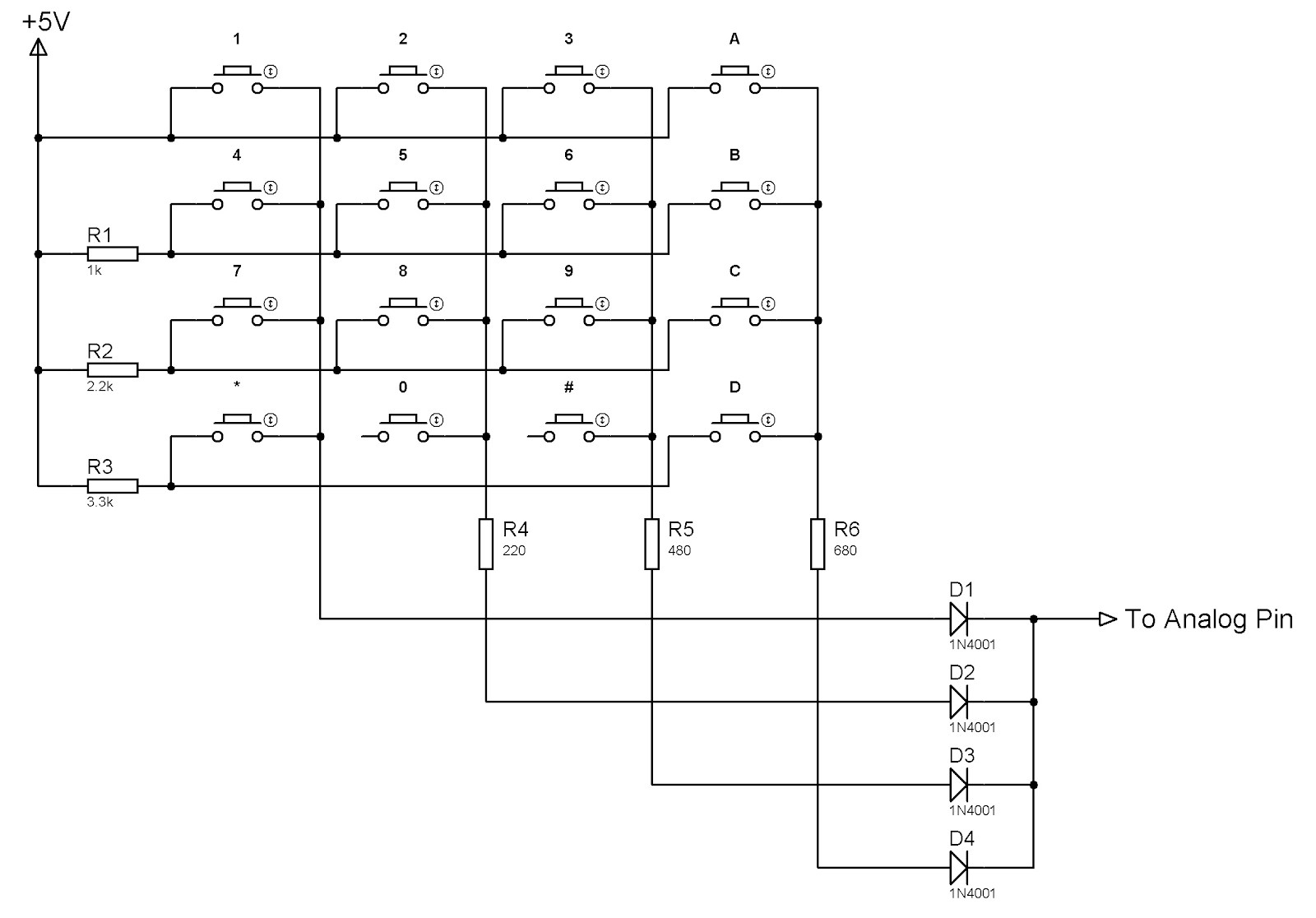Using the schematic above, when a button is pressed, a different voltage level is read on the output pin. For example, if button “4” is pressed, current will flow from the +5V source, through the 1k resistor and through the diode. The voltage at the output will be

If button “8” is pressed, the current will flow through the 2.2K and 220 resistor, giving a different voltage at the output and so on.

The downside of using this method is you have to test each button and acquire their output voltage as computations will be different with the actual value. Use the built-in AnalogReadSerial example (Files > Examples > 01.Basics > AnalogReadSerial) for testing while connecting the output pin in the schematic above to A0.

Using a 4x3 Matrix

If you own the 4x3 keypad matrix instead of the 4x4 featured in this tutorial, you can still use the same example sketches presented here. Just remove the fourth column!

I hoped you learned something from this Arduino Keypad tutorial. For updates on more tutorials like this, subscribe to this website (on the sidebar) or keep checking out this website! Have fun building!

This site uses Akismet to reduce spam. Learn how your comment data is processed.